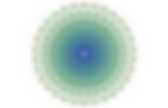# Math

Topology and Geometry. MathPuzzle.com. Math Gems. Figures for &quot;Impossible fractals&quot;. Figures for "Impossible fractals" Cameron Browne Figure 1.Making Math Fun Download Page. Differential Equations. Dimensions Home. A film for a wide audience!Nine chapters, two hours of maths, that take you gradually up to the fourth dimension. Mathematical vertigo guaranteed! Background information on every chapter: see "Details". Click on the image on the left to watch the trailer ! (turn your speakers on please). Free download and you can watch the films online! The film can also be ordered as a DVD. This film is being distributed under a Creative Commons license. Now with even more languages for the commentary and subtitles: Commentary in Arabic, English, French, German, Italian, Japanese, Spanish and Russian. Uses of other bases than 10. Math and Music – Equations and Ratios. Previously in the “math and music” lesson we derived equations for expressing intervals as functions of relative frequencies.This week we’re going to define conventions for interval sizes and then derive three variables where we can determine the composition of any frequency ratio. Guess what – all intervals can be described as different combinations of the octave, perfect fifth and major third – the first three overtones. In the last lesson we talked about the frequency ratios of common intervals. Articles — CODEE. Agent-Based Fabric Modeling Using Differential Equations by Joseph Rusinko, Hannah Swan published November 25, 2012 We use an agent-based modeling software, NetLogo, to simulate fabric drape by applying a modified mass spring system.This model provides an application of harmonic motion to textiles and fashion, fields not typically discussed in the undergraduate differential equations classroom. Euler's method is coded into the model to solve a system of ordinary differential equations describing the fabric's position over time. The On-Line Encyclopedia of Integer Sequences® (OEIS®) Mathematics WWW Virtual Library. Xah Code.

Math. Manifold. The surface of the Earth requires (at least) two charts to include every point.Here the globe is decomposed into charts around the North and South Poles. The concept of a manifold is central to many parts of geometry and modern mathematical physics because it allows more complicated structures to be described and understood in terms of the relatively well-understood properties of Euclidean space. Manifolds naturally arise as solution sets of systems of equations and as graphs of functions. Manifolds may have additional features. One important class of manifolds is the class of differentiable manifolds. Motivational examples Circle Figure 1: The four charts each map part of the circle to an open interval, and together cover the whole circle. The top and right charts overlap: their intersection lies in the quarter of the circle where both the x- and the y-coordinates are positive. Figure 2: A circle manifold chart based on slope, covering all but one point of the circle.

Paul Nylander’s Web Site. Alan Schoen geometry. Math Help. An Engineers Quick References to Mathematics Algebra Help Math SheetThis algebra reference sheet contains the following algebraic operations addition, subtraction, multiplication, and division. It also contains associative, commutative, and distributive properties.

There are example of arithmetic operations as well as properties of exponents, radicals, inequalities, absolute values, complex numbers, logarithms, and polynomials. This sheet also contains many common factoring examples. Calculus Online Book. Fundamental Theorem of Calculus, proof way. Mathematic Tutorials. Pauls Online Math Notes. Info. « hd fractals. Mathematical and Generative Graphics. Scilab Home Page. Introduction to Scilab. Terence Leung Ho Yin, Tsing Nam Kiu Table of Contents About Scilab Installing and Running Scilab Documentation and Help Scilab Basics Common Operators Common Functions Special Constants The Command Line Data Structures Strings Saving and Loading Variables Dealing with Matrices Entering Matrices Calculating Sums Subscripts The Colon Operator Simple Matrix Generation Concatenation Deleting Rows and Columns Matrix Inverse and Solving Linear Systems Entry-wise operations, Matrix Size The Programming Environment Creating Functions Flow Control Some Programming Tips Debugging Plotting Graphs 2D Graphs 3D Surfaces Scilab versus Matlab References.SCILAB. Octave. GNU Octave is a high-level interpreted language, primarily intended for numerical computations.It provides capabilities for the numerical solution of linear and nonlinear problems, and for performing other numerical experiments. It also provides extensive graphics capabilities for data visualization and manipulation. Octave is normally used through its interactive command line interface, but it can also be used to write non-interactive programs. The Octave language is quite similar to Matlab so that most programs are easily portable. LaTeX Symbols. From AoPSWiki This article will provide a short list of commonly used LaTeX symbols.MathIM: A LaTeX web-based chat. Detexify LaTeX handwritten symbol recognition. Want a Mac app?Lucky you. The Mac app is finally stable enough. See how it works on Vimeo. Download the latest version here. Restriction: In addition to the LaTeX command the unlicensed version will copy a reminder to purchase a license to the clipboard when you select a symbol. You can purchase a license here: Buy Detexify for Mac If you need help contact mail@danielkirs.ch. Web Resources. Wolfram. Mathematical Assistant on Web (main) Mathematica Online Integrator. Wolfram MathWorld: The Web's Most Extensive Mathematics Resource.